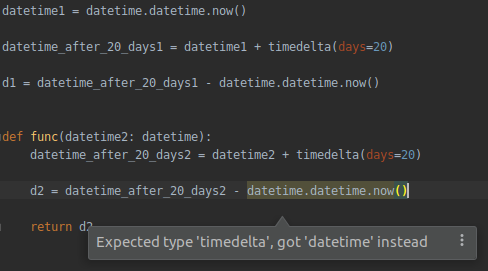Here's a sample code that I'm having a problem with.

The code just above function and in the function is the same except for variable names.I'm not sure why PyCharm is giving a warning for the same lines of code within the function but when not in function.

Here's the code in text format for the reference.

`datetime1 = datetime.datetime.now()datetime_after_20_days1 = datetime1 + timedelta(days=20)d1 = datetime_after_20_days1 - datetime.datetime.now()def func(datetime2: datetime):    datetime_after_20_days2 = datetime2 + timedelta(days=20)    d2 = datetime_after_20_days2 - datetime.datetime.now()    return d2`
1 commentHi,

Yes, the issue seems to exist and I've reported it to our tracker just in case: https://youtrack.jetbrains.com/issue/PY-39495

Meanwhile, you can resolve the issue by refactoring the code, see the example below:

`from datetime import datetime, timedeltanow = datetime.now()now_plus_20 = now + timedelta(days=20)d1 = now_plus_20 - datetime.now()def func(_now: datetime):    _now_plus_20 = _now + timedelta(days=20)    d2 = _now_plus_20 - datetime.now()    return d2`